# Remarks on Moduli Spaces and String Theory

The concept of moduli space is of utmost relevance in theoretical physics and mathematics, especially in geometry, topology and string theory. A moduli space is, roughly speaking, a space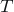that classifies a given class of objects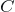modulo an equivalence relation # which identifies the objects inthat we want to consider as equivalent or, more plainly put, that we want to consider as being “the same” 12. Ideally, each point inshould correspond to an object inunique up to the given equivalence #. In other words, if we have a moduli spaceassociated to a given classification problem defined byand #, we should be able to establish a bijection betweenand the class of objectsmodulo the chosen equivalence relation #. It is in this sense that T classifies inequivalent objects in. The previous bijection identifiesand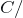as sets. However, moduli theory, namely the theory that is concerned with the study of moduli spaces, attempts to go beyond a set-theoretic identification, and, at least in a geometric context, aims at endowingwith natural geometric structures inherited from the geometric properties of the objects that we attempt to classify 3. In fact, understandingcan potentially inform on the properties of the objects we want to classify. More formally, the standard ingredients of a moduli problem are as follows:

– A class of objectsto be classified.
– An equivalence relation # on.
– A notion of family of objects inwith base space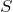, which roughly speaking is nothing but a collection of objects inparametrized by.
– A notion of equivalence of families.

These ingredients are required to satisfy a number of consistency conditions:

– A family parametrized by a single point is just an object in, and equivalence of families over a point coincides with the equivalence of objects ingiven by the relation #.
– Families behave naturally and functorially with respect to pull-backs induced by maps of base spaces.

In this note, we focus on the type of moduli problems that arise in differential geometry and related areas of string theory. A standard moduli problem in this differential-geometric context commonly considers the following set-up 4:

– A manifold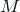fixed up to diffeomorphism. This is the underlying structure of the moduli problem.
– An additional class of structures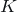of a certain type on, such as a complex structures, symplectic structures or Ricci flat metrics. The structureis usually (in interesting cases) non-unique, that is, we can endowwith many inequivalent structuresof the same type.

In this situation, one is interested in classifying all inequivalent structuresof the given chosen type. The set of all structures of typedefines the classof objects we want to classify. The notion of equivalence # depends on the moduli problem under consideration, but, for the examples mentioned above, equivalence is defined in terms of diffeomorphisms of. That is, two structures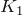and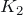are considered as equivalent if and only if there exists a diffeomorphism ofrelatingand(through its induced action on).

The classification of complex structures on a given manifold was in fact one of the first examples of moduli problems to be systematically studied, and this case greatly motivated the development of the theory of moduli spaces . A standard example of moduli problem concerning complex structures consists in classifying complex tori modulo isomorphism of complex manifolds, namely modulo biholomorphism. A complex torus is a pair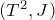consisting on a torus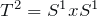and a complex structure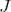on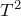. Since all torus are diffeomorphic, we can think of this classification problem as the problem of classifying all inequivalent complex structures on a given, fixed, torus. The equivalence relation is now the natural one: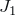and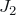are said to be equivalent if they are mapped to each other by a diffeomorphism, that is, if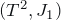and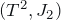are biholomorphic complex manifolds. In this situation, it can be seen that there exists an uncountable infinity of inequivalent complex tori, which are classified by the space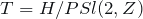, where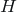denotes the upper half plane and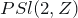acts onby fractional transformations. The famous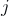-invariant gives now a biholomorphism betweenand the complex plane. In other words, points of, are in one-to-one correspondence with isomorphism classes of complex tori.

A slightly more general type of moduli problem, again in a differential-geometric context of relevance in string theory, considers the following starting set-up 5:

– A manifoldfixed modulo diffeomorphism.
– A system of partial differential equations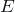on.
– A configuration space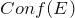for the system of partial differential equations, which corresponds with the space of variables of. Usuallycan be understood as the space of smooth sections of certain fiber bundles and can be endowed with the structure of an infinite dimensional manifold (possibly after completion).

We note that in order to properly define, it may be necessary to fix a number of extra structures on M, such as principal bundles or gerbes. This type of moduli problem is particularly relevant in the context of string theory compactifications from the space-time perspective, namely through its low-energy supergravity approximation. Given the set up introduced above, the goal is to study the moduli space of solutions of, which is defined as the space of solutions modulo the appropriate notion of equivalence. An example of this type of moduli space, which is physically motivated and had a tremendous impact in the understanding of four-manifold topology, is given by the moduli space of instantons on a closed four-dimensional Riemannian manifold. The latter consists of a particular type of solutions to the Yang-Mills equations. Yang-Mills theory was introduced by Chen Ning Yang and Robert Mills in 1954 as a mathematical model for the strong interaction. Since then, it has found impressive applications. For instance, the complete standard model of particle physics is a particular type of matter-coupled Yang Mills theory. Their classical formulation is based on the theory of connections on principal bundles, which are required to satisfy a particular partial differential equation 6.

The simplest model is given by the Yang Mills equations on an oriented manifold: a connection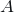on a principal bundle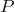satisfies the Yang Mills equations if and only if the exterior covariant derivative of the Hodge dual of the curvature ofvanishes. In four Riemannian dimensions there is a particular class of connections, called (anti) self-dual instantons, that automatically satisfy the Yang Mills equations. An (anti) self-dual instanton on an oriented four manifoldis a connection on a principal bundleoverwhose curvature is self-dual or anti self-dual. On a closed manifold, these instantons are absolute minimizers of the Yang-Mills functional, whence it is natural to study the moduli space of instantons onmodulo the appropriate notion of equivalence. The appropriate notion of equivalence of instantons, or connections in general, corresponds in this context with gauge equivalence, that is, two connections onare equivalent if they are related by a gauge transformation, which is nothing but a based automorphism of. The (anti) self-duality condition is an elliptic condition modulo gauge transformations, whence it is reasonable to expect that the moduli space of instantons over a closed manifold M be finite dimensional. This is indeed the case, and when appropriately defined, the moduli becomes a particular type of orbifold. From the mathematical point of view, the main motivation for studying the moduliof instantons is that it carries crucial information about the topological properties of M as a smooth manifold. By a careful study of, Simon K. Donaldson was able to prove in 1983 the following outstanding result 7:

Theorem: Letbe a simply connected closed four manifold with positive-definite intersection form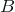. Then,is diagonalizable.

Shortly before, Michael H. Freedman 8 had proven that every unimodular symmetric bilinear form is realized as the intersection form of a simply connected closed topological four manifold. As there are plenty of unimodular symmetric bilinear forms which are not diagonalizable over the integers, the previous theorem implies that the simply connected topological four-manifolds which realize these non-diagonalizable bilinear forms do not admit smooth structures. The question whether every simply connected closed four manifold admits a differentiable structure was indeed an important open problem in topology. After this theorem appeared there was an explosion of activity in the exploration of moduli spaces of instantons and their applications to four manifolds. A new area of geometry and topology was born: mathematical gauge theory. Only a few years later, in 1994, Edward Witten shocked the community by proposing a set of invariants, the Seiberg-Witten invariants, which were conjectured to contain the same information as the polynomial Donaldson invariants while being based on a much simpler theory inspired by supersymmetry 9. This theory is Seiberg-Witten theory, and remains to these days as one of the outstanding applications of supersymmetry to mathematics.

Aside from their mathematical applications, moduli spaces can also play a crucial role in the formulation of certain physical theories, such as the usual suspect, a.k.a. the only game in town, a.k.a. string theory . In order to obtain four-dimensional physical models, string theory is usually compactified on an appropriate closed manifold, for example a closed Calabi-Yau three-fold or a closed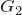holonomy seven dimensional manifold (the latter in the case of M-theory) 10. From the space-time point of view, the effective theory describing such compactifications is a supergravity theory preserving a given number of supersymmetries. The number of preserved supersymmetries depends on the topological and geometric details of the internal compactification manifold.

Supergravity theories are supersymmetric theories of gravity whose mathematical global structure is constrained in a beautiful and delicate way by supersymmetry. The global mathematical structure of supergravity is still far from being completely understood, although an impressive amount of results has been obtained during the last four decades regarding its local structure. It is known that Supergravity theories contain non-linear sigma models whose target spaces are given by Riemannian manifolds carrying special geometric structures. For instance, the target space of the sigma model of four-dimensional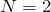supergravity is locally given by a product of a projective Special-Kähler manifold and a Quaternionic-Kähler manifold. Similarly, the target space of the maximally supersymmetric supergravity theory in four dimensions, namely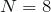supergravity, is, interestingly enough, locally isometric to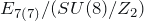. Here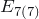denotes the maximally non-compact real form of the complex exceptional Lie group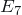and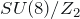denotes its maximal compact subgroup. The remarkable fact here is that supersymmetry is believed to imply that these local geometries should precisely correspond to the local moduli space of the compactification that yields the given lower-dimensional supergravity theory. From the higher-dimensional supergravity point of view, compactification moduli spaces correspond to the moduli space of solutions to the supergravity equations of motion on the internal compact space. These moduli spaces usually include as a subset the moduli space of interesting geometric structures of intrinsic mathematical relevance, such as the moduli space of torsion-free Calabi-Yau orstructures. Since supergravity equations are very involved, supergravity moduli spaces are in general incredibly hard to characterize. Therefore, it is remarkable that low-dimensional supergravity can potentially predict the geometry of compactification moduli spaces just from its supersymmetric structure. The theory of moduli spaces in geometry and topology has been a crucial ingredient of string theory since its very beginning, in the long tradition of this theory/framework of containing remarkably rich connections with state of the art mathematics. Despite their importance and an increasing activity in the topic, most of the mathematical problems related to supergravity theories and their compactification moduli spaces remain completely open.

## References

1. P. E. Newstead , Introduction to Moduli Problems and Orbit Spaces, A publication of the Tata Institute of Fundamental Research.
2. Marco Manetti , Lectures on deformations of complex manifolds, Rendiconti di Matematica 24, (2004), 1-183.
3. Atiyah, M., Bott, R.: The Yang-Mills equations over a Riemann surface. Phil. Trans. R. Soc A308 523 (1982).
4. Kodaira, Kunihiko, Complex Manifolds and Deformation of Complex Structures, Springer-Verlag (2005).
5. S. K. Donaldson and P. B. Kronheimer, The Geometry of Four-Manifolds, Oxford Mathematical Monographs (1997).
6. K.B. Marathe and G. Martucci, The Mathematical Foundations of Gauge Theories, North Holland (1992).
7. Donaldson, Simon K., “An application of gauge theory to four-dimensional topology”. J. Differential Geom. 18 (2): 279–315 (1983).
8. Michael H. Freedman and Frank Quinn, Topology of 4-manifolds, Princeton Mathematical Series, 1990.
9. W. Lerche, Introduction to Seiberg-Witten Theory and its Stringy Origin, Nucl.Phys.Proc.Suppl. 55B (1997) 83-117; Fortsch.Phys. 45 (1997) 293-340.
10. Katrin Becker,Melanie Becker and John H. Schwarz, “String Theory and M-Theory: a Modern Introduction”. January 2007. Cambridge University, Cambridge University Press, 2007.